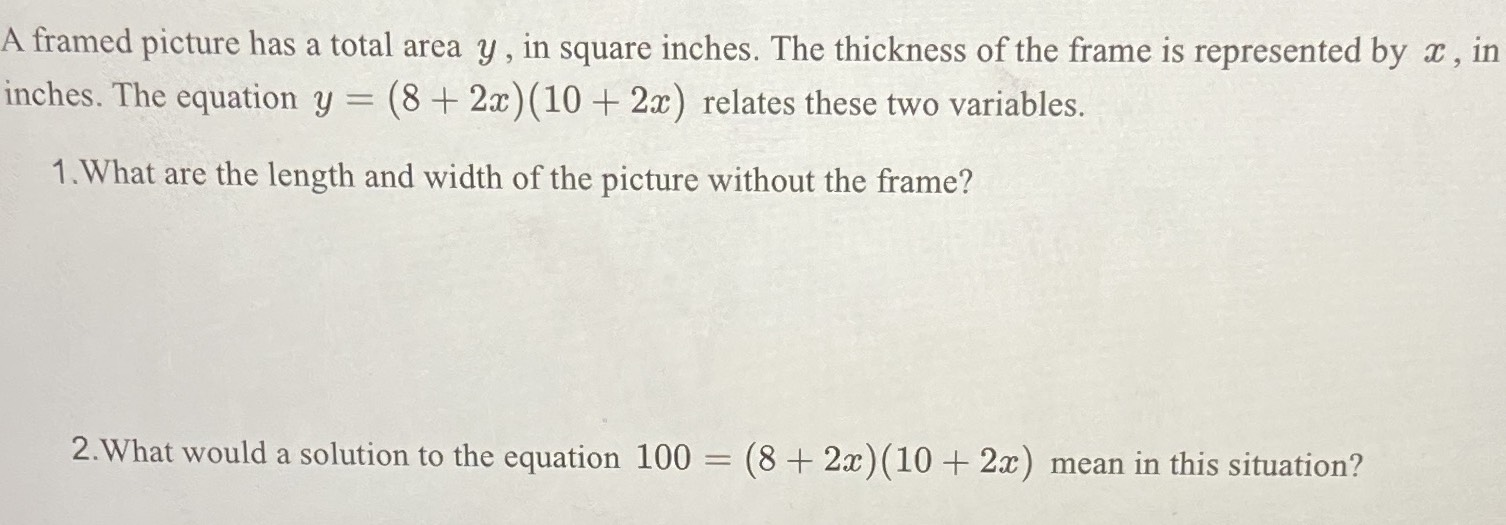### ¿Todavía tienes preguntas de matemáticas?

Pregunte a nuestros tutores expertos
Algebra
PreguntaA framed picture has a total area $$y$$ , in square inches. The thickness of the frame is represented by $$x$$ , in inches. The equation $$y = ( 8 + 2 x ) ( 10 + 2 x )$$ relates these two variables.

1. What are the length and width of the picture without the frame?

2. What would a solution to the equation $$100 = ( 8 + 2 x ) ( 10 + 2 x )$$ mean in this situation?

$$100= \left(8+ 2x\right)\left(10+ 2x\right)\quad : \quad x= \frac{- 9+ \sqrt{101}}{2},\space x= -\frac{9+ \sqrt{101}}{2}$$
2x+8=-1+$$\sqrt{101}$$
2x+10=1+$$\sqrt{101}$$